# CBSE Class 9 Science Force And Laws Of Motion Notes Set B

FORCE AND LAWS OF MOTION

FORCE

Consider a ball kept on a table, we can move it by pulling or pushing. We can increase its speed by pushing it in the direction of motion. If we push it opposite to the direction of motion its speed will decrease. If the ball is in motion towards east, we push it towards north, the direction of will change.

Take a soft rubber ball between your palms and push the ball from both sides, the shape of the ball is distorted. In all the above cases we have applied the force on the ball.

So force in a push or pull which can move the object. It can change the speed of the object, it can change the direction of motion, it can change the shape of the object. In all the above cases we have applied the force on the ball and the ball is accelerated so we can define force as follows :

“Force is the cause which can produce acceleration in the body on which is acts”.

(a) Effects of Force :

The force or a set of forces acting on a body, can do three things :

(i) A force or a set of forces can change the speed of the body.

(ii) A force or a set of forces can change the direction of motion.

(iii) A force can change the shape of the body.

GALILEO’S EXPERIMENTS

Experiment 1 :

It was observed by Galileo that when a ball is rolled down on an inclined plane it speed increases, whereas if it is rolled up an inclined plane its speed decreases. If it is rolled on a horizontal plane the result must be between the cases describe above i.e. the speed should remain constant. If can be explain as -Experiments 2 :

When a ball sin released on the inner surface of a smooth hemisphere, it will move to the other side and reach the same height before coming to rest momentarily. If the hemisphere is replaced by a surface shown in figure (b) in order to reach the same height ht ball will have to move a larger distance.if the other side is made horizontal, the ball will never stop because it will never be able to reach the same height, it means its speeds will not decrease. It will have uniform velocity on the horizontal surface. Thus, if unbalances forced do not act on a body, the body will either remain at rest or will move with a uniform velocity. It will remain uncelebrated.

(a) Conclusion of Galileo’s Experiments :

(i) A body is at rest and no unbalanced forced acts on it, remains at rest.

(ii) A body is moving and no unbalance force acts on it, it will continue to move at   constant speed in a fixed direction.

(iii) If unbalance forces act on a body the body will accelerated. The idea was suggested by Galileo and was later formulated into laws by Newton.

NEWTON’S FIRST LAW OF MOTION

We have learnt so far that :

(i) If a body is at rest and no unbalanced force acts on it, it remains at rest.

(ii) If a body is moving and no unbalanced force acts on it, it will continue to move at constant speed in a fixed direction.

(iii) If an unbalanced force acts on a body, the body will accelerated.

These facts are taken together from Galileo’s law of inertia on Newton’s’ first law of motion. The idea was suggested by Galileo and was later formulated into a law by Newton. We can state Newton’s first law motion as follows :

(a) Statement of Newton’s First law of Motion :

A body at rest will remain at rest and a body in motion will remain in uniform motion unless acted upon by an unbalanced force.

This law also be stated as follows :

A body remain uncelebrated if and only if, the resultant force on it is zero.

In such a case the body is said to be in equilibrium.

INERTIA

Description :

If follows from first law of motion that is absence of any eternal force, a body continues to be in its state of rest or of uniform motion along a straight line. In other words, the body cannot change by itself its position of rest or of uniform motion.

The inability of the body to change by itself its states of rest or uniform motion is a straight line is called inertia. Newton’s’ first law of motion is also called law of inertia.

(a) Inertia Depends upon Mass :L

We know that it is difficult to move a heavier body than the lighter one. Similarly it is difficult to stop a moving heavier body that  a lighter body moving with the same velocity. Thus, we conclude that mass of the body is the measure of inertia, more the mass, more the inertia.

TYPES OF INERTIA

Inertia is of the three types :

(a) Inertia of Rest :

The tendency of the body to continue is state of rest even when some external unbalance force is applied on it, is called the inertia of rest.

Description :

(i) A person sitting in a bus falls backwards when the bus suddenly starts. The reason is the lower part of his body begins to move along with the bus but the upper part of his body tends to remain at due to inertia of rest.(ii) We beat a carpet with a stick to remove dust particles. When the carpet is beaten, it is suddenly set into motion. The dust particles tend to remain at rest due to inertia of rest and hence fall off.

(iii) When a branch of tree is shaken the fruits get separated from the tree due to inertia of rest.

(b) Inertia of Motion :

The tendency of the body to continue in its state of motion even when some unbalance forces are applied on it, is called in the inertia of motion.

Description :

(i) A man carelessly getting down a moving bus falls forward, the reason being that his feet come to rest suddenly, whereas the upper part of his body retains the forward motion.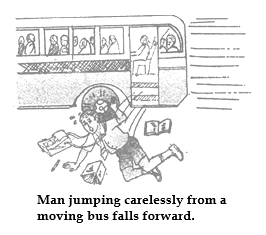(ii) An athlete runs a certain distance before taking a leap so that the inertia of motion of his body at the time of leaping may help him in his muscular efforts.

(iii) We remove snow or mud from our shoes by striking them against wall. On striking the wall, the feet comes to rest whereas the snow which is still in motion separates from the shoes.

(c) Inertia of Direction :

The tendency of a body to oppose any change in its direction of motion is known as inertia of direction.

(i) If a car takes a turn along a curved track, the passengers experience a force acting away from the centre of the curved track. This is the result of tendency of the passenger to continue moving along a straight path.

(ii) Tie a stone to one end of a string and holding other end of the string in hand. rotate the stone in a horizontal circle. if during rotation, the string breaks at certain stage, the stone is found to fly off tangentially at that point of the circle.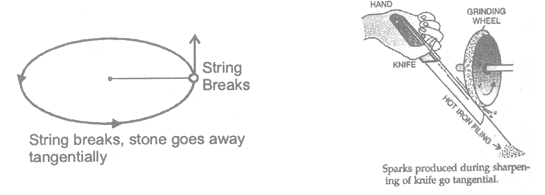(iii) The water drops sticking to cycles tyre are found to fly off tangentially.

(iv) The sparks produced during sharpening of a knife or a razor against a grinding wheel, leave the rim of the wheel tangentially.

DEFINITION OF FORCE FROM FIRST LAW OF MOTION

Description :

A according to first law of motion, if there is no force, there is no change in state of rest or of uniform motion. In other words, if a force is applied, it may change the state of rest or of uniform motion. If the force is not sufficient, it may not produce a change but only try to do so.

Hence force is that which changes or tries to change the state of rest or of uniform motion of a body in straight line.

MOMENTUM

Description :

It is the combined effect of mass and velocity of the body. Mathematically, momentum of the body is defined as the product of mass and the velocity of the body. If m is the mass of the body and v is its velocity then momentum, p = mv

Momentum is a vector quantity and its direction is in the direction of velocity.

Unit of momentum :

(In C.G.S. system) → p = mv  gram × cm/s = dyne × s

(In M.K.S. system)  p = mv  kg ×  m/s = Newton × s

NEWTON’S SECOND LAW OF MOTION

The rate of change of momentum of a body is directly proportional to the applied unbalanced forces i.e. Rate of change of momentum  Force applied

Let a body is moving with initial velocity u and after applying a force F on it, its velocity becomes v in time t.

Initial momentum of the body p1 = mu

Final momentum of the body p2 = mv

Change in momentum in time t is mv - mu

So rate of change of momentum =mv - mu / t

But according to Newton’s second law, mv - mu / t α F

Or   α m(v-u)  /t    Here, v-u/ t=a   (acceleration)

So    F α   ma

or     F = kma  Here is proportionality constant.

if 1N force is applied on a body of mass 1 kg and the acceleration produced in the body is 1 ms/2, then 1 = k × 1 × 1 or k = 1.

So the magnitude of the resultant force acting on body is equal to the product of mass of the body and the acceleration produced. Direction of the force is same as that of the acceleration.

UNITS OF FORCE

(a) In C.G.S. System :

F = ma  gm × cm/s2 = Dyne

Definition of one dyne :

If m = 1 gm, a = 1 cm/s2, then F = 1 dyne.

When a force is applied on a body of mass 1 gram and the acceleration produced in the body in 1 cm/s2 then the force acting on the body will be one dyne.

(b) In S.I. System :

F = ma  kg × m/s2 = Newton

Definition of one Newton :

If m = 1 kg and a = 1 m/s2 then by, F = ma

F = 1 × 1 = 1 kg m/s2 = 1 N.

If a force is applied on a body of a mass 1 kg and acceleration produced in the body in 1 m/s2, then the force acting on the body will be one Newton.

Other units :

There are two other units of force called gravitational units.

(c) Kilogram Force (kgf) :

Kilogram force (kf) or Kilogram weight (kg. wt.) is force with which a mass of 1 kg is attracted by the earth towards its centre.

1 kgf = 9.8 N

(d) Gram Force (gf) :

Gram force or gram weight is the force with which a mass of 1 gram is attracted by the earth towards its centre.

1 gf = 981 dyne

Relation between Newton and dyne.

We know :

1 N = 1kg = 1 ms-2

or 1 N = 1000 g × 100 cms

or 1 N = 10g cms = 105 dyne

1 N = 105 dyne

FIRST LAW OF MOTION BY SECOND LAW OF MOTION

Description :

According to first law of motion, if there is no force, there is no change in state of rest or of uniform motion. In other words, if a force is applied, it may change the state of rest or of uniform motion. If the force is not sufficient, if may not produce a change but only try to do so. Hence force is that which changes o tries to change the state of rest or of uniform motion of a body in straight line.

Hence we get the definition of force from Newton’s first law of motion.

Newton’s first law of motion can be deduced from Newton’s’ second law of motion.

According to second law of motion,

F = ma

if   F = 0, then a = 0

Since m ≠ 0

So  a= v-u / t=0

or  mv = mu

or   v - u = 0 or v = u

or   v = u              [after more time]

Which means that the velocity of the body cannot change in absence of external force. If the body is initially at rest i.e., if u = 0, v = 0 and if u = 5 ms-1, v = 5ms-1.

Thus, it follows that a body will continue to be in the state of rest or of uniform motion along a straight line if no external force acts on it and this is the first law. thus, first law can be deduced from second law of motion.

ILLUSTRATIONS

1.A force F1acting on a body of 2 kg produces an acceleration of 2.5 ms2. An other force Facting on the another body of mass 5 kg produces an acceleration of 2 m/sec2. Find the ratio .F1/F2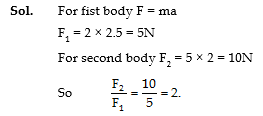2.A force of 20N acting on amass m1produces an acceleration of 4 ms-2. The same force is applied on mass m2 then the acceleration produced is 0.5 ms-2. What acceleration would the same force produce, when both masses are tied together ?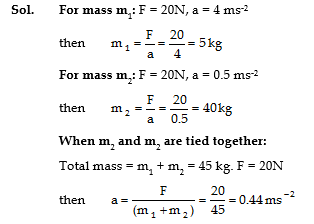IMPULSES OF FORCE

(a) Introduction :

In previous article, we leant that a moving body has momentum and that on effect (a force) is needed to stop it. It is our common experience that a smaller force takes more time to stop the body whereas a bigger force does the same in lesser time. This observation gives concept of a new quantity, force × time, which is named a impulse.

(b) Definition :

The product of the magnitude of a force applied on a  body and the time for which it is applied, is called impulse of the force. It is represented by the symbol (I).

i.e., Impulse = Force × Time

or I = F. t

The S.I. unit of impulse is Newton-second (N-s) and the C.G.S unit is dyne - second (dyne -s)

(c) Impulse and Momentum :

From Newton’s second law of motion

Force, F= p2-p1 /t           or       F.t=    p2-p1

i.e., Impulse = Change in momentum

This relation is called impulse equation or momentum-impulse theorem. It has an important application in our everyday life.

APPLICATIONS OF IMPULSE EQUATION IN DAILY LIFE

(i) Catching the ball by a cricketer :

While catching a fast moving cricket ball, the player moves his hands backward  after catching the ball. By moving his hands, the cricketer increases the time. As a result he has to apply a small force on the ball. In reaction, the ball also applies lesser force and the hands of the player are not injured.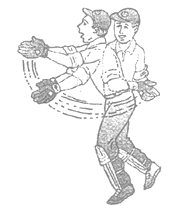(ii) Jumping on heap of  sand :

If someone jumps from a height on a heap of sand below, his feet move inside the sand very slowly. His momentum changes slowly requiring a lesser force of action from the sand. The man is not injured.

(iii) Jumping down of a passenger from a moving train or bus :

A passenger sitting in a moving train or bus has momentum, When the jumps down and stands on platform or road, his momentum becomes zero.

If he jumps down suddenly from the moving train or bus and tries to stand on his feet, his body will fall forward due to inertia of motion. He will be injured.

He is advised to run over some distance on the platform or road along with (in direction f) the train or bus. This will slow down his rate of change of momentum and lesser force will be involved.

(vi) Springs in vehicles :

The vehicles are fitted with springs to reduce the hardness of the shock. When vehicles more over an uneven road, they experience impulses exerted by the road. The springs increase the duration of impulse and hence reduce the force.

(v) Springs in seats :

The seats are also fitted with springs to reduce their hardness. When we sit on them all of a sudden, the seats are compressed. The compression increases duration of our coming to rest of the seat. They reaction force of seats become negligible.

(vi) Soft material packing :

China and glass wares are packed with soft material when transported. They collide during transportation but soft packing material slows down their rate of change of momentum. The force of impact is reduced and the items are not broken.

(vii) Atheists :

Athletes are advised to come to stop slowly after finishing a fast race. In general, all changes of momentum must be brought slowly to involve lesser force of action and reaction to avoid injury.

IMPULSE DURING AN IMPACT OR COLLISION

The impulsive force acting on the body produces a change in momentum of the body on which it acts. We know, Ft = mv - mu, therefore maximum force needed to produce a given impulse depends upon time. If time is short, the force required in a given  impulse or the change in momentum is large and vice - versa.

NEWTON’S THIRD LAW

(a) Introduction :

When a force is applied to stop a moving body, we ourselves experience some force from the body being stopped. When a cricketer used his hands to stop a moving ball, his hands also experience some force from the ball and sometimes the force is unbearable. When we jump on a cemented road from some height, our feet get injured by the impact of the road.

From above examples we find that whenever one body exerts a force on another body, the second body exerts an equal and opposite force on the first body. The force exerted by the first body on the second body is called ‘action’ and the force exerted by the second body on the first body is called ‘reaction’/

(b) Statement :

The law states the “To every action there is an equal and opposite reaction”. Moreover, action and reaction act on different bodies.

(c) Demonstration :

Two similar spring balances A and B joined by hook as  shown in the figure, The other of the spring balance B is attached to a hook rigidly fixed in a rigid wall.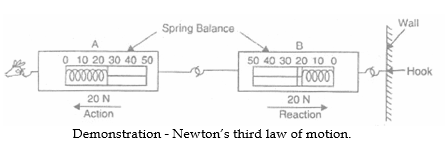The other end of the spring balance A is pulled out to the left. Both the balances show the same reading (20 N) for the force.

The pulled balance A exerts a force of 20N on the balance B. It acts as action, B pulls the balance A in opposite direction with a force of 20 N. This force is known as reaction.

We conclude that action-reaction forces are equal and opposite and act on two different bodies.

(b) Explanation :

If may be noted that action and reaction occur simultaneously. Action and reaction never act on same body. Had this been the case, there would have been no (accelerated) motion, Since action and reaction occur in pairs and act on two different bodies, it is impossible to have single isolated force.

(e) Examples :

(i) Swimming of a man : The man swims because he pushes water behind (action), water pushes man forward (reaction).

(ii) Walking of man : man pushes the earth behind from right foot (action). Earth pushes the man forward (reaction). Then the man walks.(iii) Flight of jet or rocket :The burnt gases are exhausted from behind with high speed giving the gases backward momentum (action). The exhausted gages impart the jet or rocket a forward momentum (reaction). Then jet or rocket moves.

(iv) Gun and bullet : A loaded gun has a bullet inside it. When the gun’s trigger is pressed, the powder inside cartridge explodes. A force of action acts on the bullet and makes the light bullet come out of the barrel with a high velocity. The heavy gun moves behind (recoils) with a small velocity due to force of reaction.

This is also an example of law of conservation of linear momentum.

(v) Man and boat : A man is boat near river bank is at rest. To reach the bank, the man pushes the boat behind (action), the boat pushes the man forward (reaction). The man lands on the bank.

(vi) Hose pipe : Water rushes out of the hose pipe with a large velocity due to force of action of the compressor from behind. The rushing out jet of water pushes the hose pipe behind due to force of reaction. Then pipe has to be held tightly.

NO ACTION IS POSSIBLE WITHOUT REACTION

Examples :

(i) A nail cannot be fixed on a suspended wooden ball.

(ii) A paper cannot be cut by scissors of single blade.

(iii) A hanging piece of paper cannot be cut by blade.

(iv) Writing on a hanging page is impossible.

(v) Hitting on a piece of sponge does not produce reaction. You do not enjoy hitting.

ACTION AND REACTION ARE NOT BALANCED

Action and reaction, through equal and opposite are not balanced because they act on two different bodies. If case when they act on two different bodies forming a single system, they become balanced.

INTERACTION BETWEEN BODOIS AT A DISTANCE

We have uptill now considered examples where the two bodies are in direct contact with each other but. But interaction takes place even when the two bodies are not in actual contact with each other. For example, a comb rubbed with dry hair can interact with a piece of paper from a distance. Similarly a magnet can interact with an iron piece from a distance. Interaction between a falling stone and the earth also takes place although these are not in actual contact with each other. Thus when one body influences another body by applying force with or without contact, we say that the first body is interacting with the second body.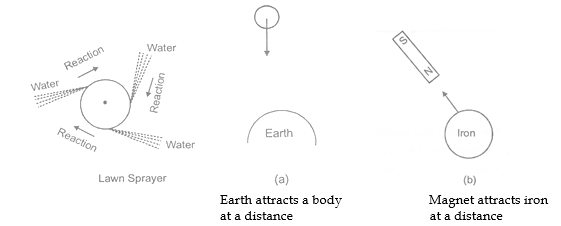ANY PAIR OF EQUAL AND OPPOSITE FORCES IN NOT AN ACTION - REACTION PAIR

Consider a book kept on a table. We have seen that the table pushes the book in the upward direction. They why does not the book fly up ? It does not fly up because there is another force on the book pulling it down. This is the force exerted by the earth of the book, which we call the weight of the book. So, there are two forces on the book-the normal force, N acting upwards, applied by the table and the force, W acting downwards, applied by the earth. As the book does not accelerate, we conclude that these two forces are balanced. In other words, they have equal magnitudes but opposite direction.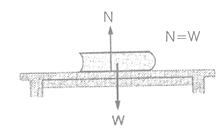Can call N the action and W the reaction ? We cannot. This is because, although they are equal and opposite, they are not forces applied by two bodies on each other. The force N is applied by the table on the book, its reaction will be the force applied by the book on the table. Weight W is the force applied by the earth on the book, its reaction will be the force applied by the book on the earth.

So, although N and W are equal and opposite, they do not form an action - reaction pair.

PRINCIPLE OF CONSERVATION OF LINEAR MOMENTUM

By Newton’s second law, the rate of change of momentum is equal to the applied force.

change in momentum / time   = Force

Change is momentum = F × t

If F = 0 then,

Change is momentum = 0

If the force applied on the body is zero then its momentum will be conserved, this law is also applicable on the system. If in a system the momentum of the objects present in the system are P1 , P2, P........... and external force on the system is zero, then -

P1 + P2 + P3 + .............. = Constant

• NOTE : If only internal forces are acting on the system then its linear momentum will be conserved.

(a) The Law of Conservation of Linear Momentum by Third Law of Motion :

Suppose A and B are two objects of masses m1 and m2 are moving in the same direction with velocity u1 and urespectively (u> u2). Object A collides with object B and after time t both move in their original direction with velocity v1 and v2 respectively.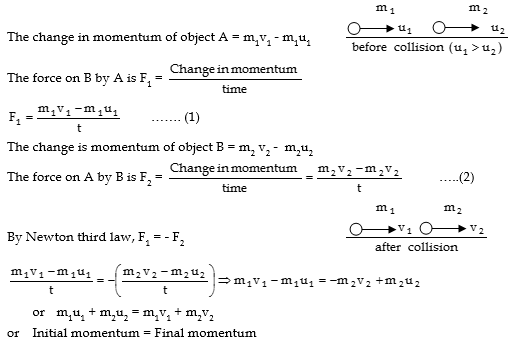SOME ILLUSTRATION ON CONSERVATION OF MOMENTUM

(a) Recoil of Gun :

A loaded gun (rifle) having bullet inside it forming one system is initially at rest. The system has zero initial momentum.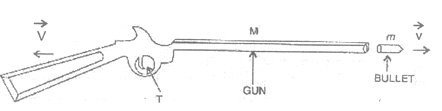When the trigger (T) is pressed, the bullet is fired due to internal force of explosion of powder in cartridge inside.

The bullet moves forward with a high velocity and the gun move behind (recoils) with a lesser velocity.

Let the bullet and the gun have masses m and M respectively. Let the bullet move forward with velocity v and the gun recoils with velocity V.

Then final momentum of the gun and bullet is MV + mv

By the law of conservation of momentum :

Initial momentum of the system = Final momentum of the system.

0 = MV + mv       or V- mv/ M

Hence the recoil velocity of gun = mv / M

and the velocity of the gun is = -mv / M

(a) Flight of Jet Rocket :

Jet planes and rockets are provided with chemical fuels. Combustion of these fuels produces a high velocity blast of hot gases. These gases move outward and escape through nozzle (a narrow opening) with very high velocity and large momentum. (they escape horizontally backward in case of jet planes and vertically downwards in case of rocket). The escaping gases impart their momentum to the jet plane and the rocket. They move forward or upward with a high velocity.

In general, all cases involving action and reaction, are examples of law of conservation of momentum. Action and reaction being equal and acting simultaneously for same duration, have equal and opposite impulses. They produce equal and opposite changes of momentum in the pair of bodies involved. It keeps the total momentum of the two body system constant (conserved).

ILLUSTRATIONS

1. A field gun a mass 1.5 t fires a shell of mass 15 kg with a velocity of 150 m/s. Calculate the velocity of the recoil of the gun.

Sol.Mass of gun = 1.5 t = 1.5 × 1000 kg = 1500 kg

Mass of shell = 15 kg

Velocity of shell = 150 m/s.

Velocity of recoil of the gun = ?

Momentum of gun = Mass of gun × velocity of recoil of the gun = 1500 V kg m.s

Momentum of shell = Mass of shell × velocity of shell = 15 × 10 kg m/s.

By the law of conservation of momentum :

Momentum of gun = Momentum of shell

1500 V = 15 × 150    or    V =15 × 150 / 1500= 1.5 m/s

The recoil velocity of gun = 1.5 m/sec.

1. A hunter of 45 kg is standing on ice fires a bullet on 100 gram with a velocity of 500 ms-1 by a gun of 5 kg. Find the recoil velocity of the hunter.

Sol.  The initial momentum of the system, P= Momentum of hunter + momentum of gun  + momentum of bullet

or P1 + 45 × 0 + 5 × 0 + 0.1 × 0 = 0                ........... (1)

Final momentum of the system, P1 = Momentum of hunter + Momentum of gun + momentum of bullet

P= 45 V + 5 V + 0.1 × 500             (Here V is the recoil velocity of gun with hunter).

P2 = 50 V + 50 ......(2)

By the conservation of momentum

P1 = P2

0 = 50 V + 50       or            V = -1 m/s.

The recoil velocity of gun with hunter is 1 m/s.

## Tags:

Click for more Science Study Material

## Latest NCERT & CBSE News

Read the latest news and announcements from NCERT and CBSE below. Important updates relating to your studies which will help you to keep yourself updated with latest happenings in school level education. Keep yourself updated with all latest news and also read articles from teachers which will help you to improve your studies, increase motivation level and promote faster learning

### Board Cancelled Official CBSE Statement

Keeping in view the requests received from various State Governments and the changed circumstances as on date, following has been decided- 1. Examinations for classes X and XII which were scheduled from 1st July to 15th, 2020 stand cancelled. 2. Assessment of the...

### Internal Assessments and CBSE Reduced Syllabus

CBSE on 7th July 2020 had announced the ‘Revised Academic Curriculum for classes IX-XII for the session 2020-21’ to mitigate the effect of face to face learning loss due to the closure of schools to contain COVID-19 Pandemic. The rationalization of syllabus up to 30% ...

### FAQs CBSE Board Exam Results Class 10 and 12

FAQs CBSE Board Exam Results Class 10 and 12 Q.1. What does the term RT in the marksheet mean? Ans.The term RT means REPEAT IN THEORY. This is the term used from 2020 instead of FAIL IN THEORY(FT) Q.2. What does the term RP in the marksheet mean? Ans.The term RP means...

### CBSE Reduced Syllabus Clarification

CBSE board has issued the following clarification relating to various news reports regarding reduction in syllabus and questions from the reduced syllabus during board exams. Please read below: It has come to notice of the Board through a section of media reports that...

### Re verification of Class 12 Board Exams Marks

Modalities and Schedule for Senior Secondary School (Class XII) Examinations, 2020 in the subjects whose examinations have been conducted by CBSE for the processes of (I) Verification of Marks (II) Obtaining Photocopy of the Evaluated Answer Book(s) (Ill) Re-evaluation...

### Re verification of Class 10 Board Exams Marks

Modalities and Schedule for Secondary School (Class X) Examinations, 2020 in the subjects whose examinations have been conducted by CBSE for the processes of (I) Verification of Marks (II) Obtaining Photocopy of the Evaluated Answer Book(s) (Ill) Re-evaluation of Marks...

×